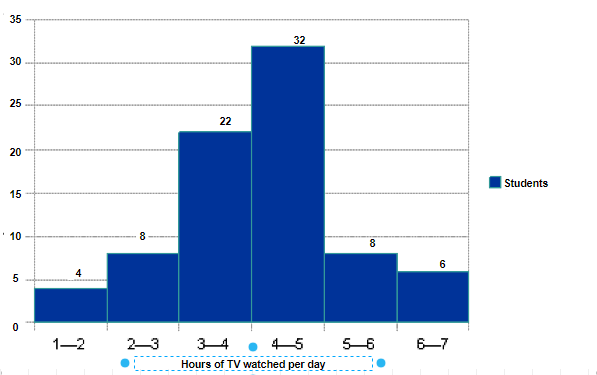# Ex.5.1 Q5 Data Handling Solutions - NCERT Maths Class 8

Go back to  'Ex.5.1'

## Question

The number of hours for which students of a particular class watched television during holidays is shown through the given graph. We draw the histogram for above frequency table:(i) For how many hours did the maximum number of students watch T.V.?

(ii) How many students watched TV for less than $$4$$ hours?

(iii) How many students spent more than $$5$$ hours in watching TV?

Video Solution
Data Handling
Ex 5.1 | Question 5

## Text Solution

What is known?

Histogram representing the number of students versus hours of TV watched per day.

What is unknown?

(i) Number of hours did the maximum number of students watch T.V.

(ii) Number of students watched TV for less than $$4$$ hours.

(iii)Number of students spent more than $$5$$ hours in watching TV.

Reasoning:

Histogram is a graphical representation of data, the data represented in manner of class- interval.

Steps:

(i) The maximum number of students watched T.V. for $$4 – 5$$ hours.

(ii) $$34$$ students watched T.V. for less than $$4$$ hours.

(iii) $$14$$ students spent more than $$5$$ hours in watching T.V.

Learn from the best math teachers and top your exams

• Live one on one classroom and doubt clearing
• Practice worksheets in and after class for conceptual clarity
• Personalized curriculum to keep up with school